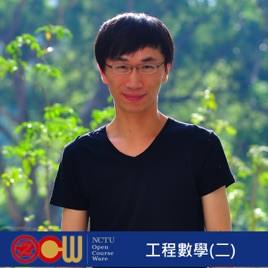36 個單集

This lecture aims to provide the students with an integrated knowledge required for the understanding and application of mathematical concepts and techniques. The emphasis will be on the application of mathematical methods to solving practical engineering problems. Upon satisfactory completion of the subject, students are expected to be able to:
(i) apply mathematical reasoning to analyze essential features of different engineering problems;
(ii) apply appropriate mathematical techniques to model and solve problems in engineering;
(iii) search for useful information in solving problems;
(iv) undertake continuous learning.# 工程數學(二) Engineering Mathematics(II) -102學年度 National Chiao Tung University

• 教育

This lecture aims to provide the students with an integrated knowledge required for the understanding and application of mathematical concepts and techniques. The emphasis will be on the application of mathematical methods to solving practical engineering problems. Upon satisfactory completion of the subject, students are expected to be able to:
(i) apply mathematical reasoning to analyze essential features of different engineering problems;
(ii) apply appropriate mathematical techniques to model and solve problems in engineering;
(iii) search for useful information in solving problems;
(iv) undertake continuous learning.

• video
矩陣之基本介紹與定義

## 矩陣之基本介紹與定義

• 37 分鐘
• video
各種矩陣型式之介紹與線性代數系統之矩陣應用

## 各種矩陣型式之介紹與線性代數系統之矩陣應用

• 41 分鐘
• video
線性代數系統之矩陣應用, 矩陣之階次

• 52 分鐘
• video
矩陣之線性系統

• 35 分鐘
• video
矩陣之行列式與倒數

• 41 分鐘
• video
矩陣之倒數

• 36 分鐘